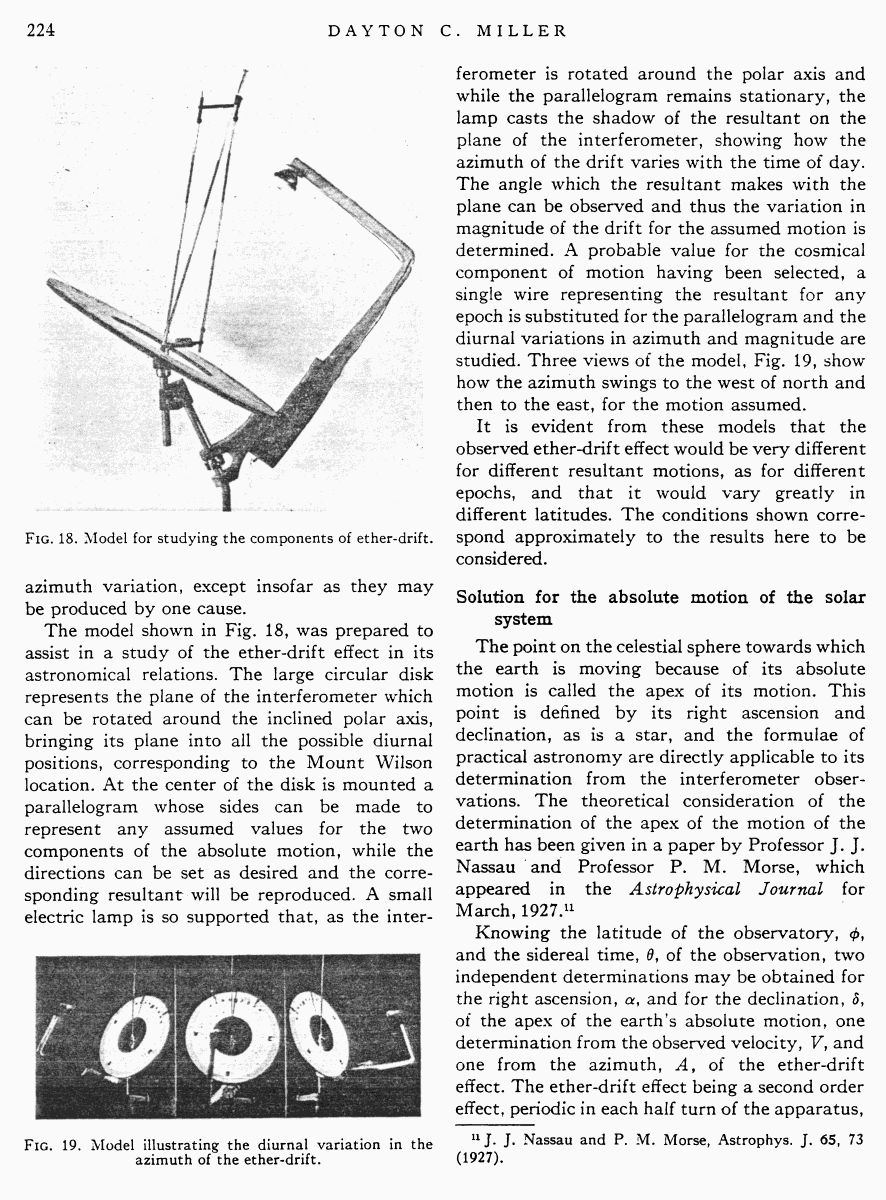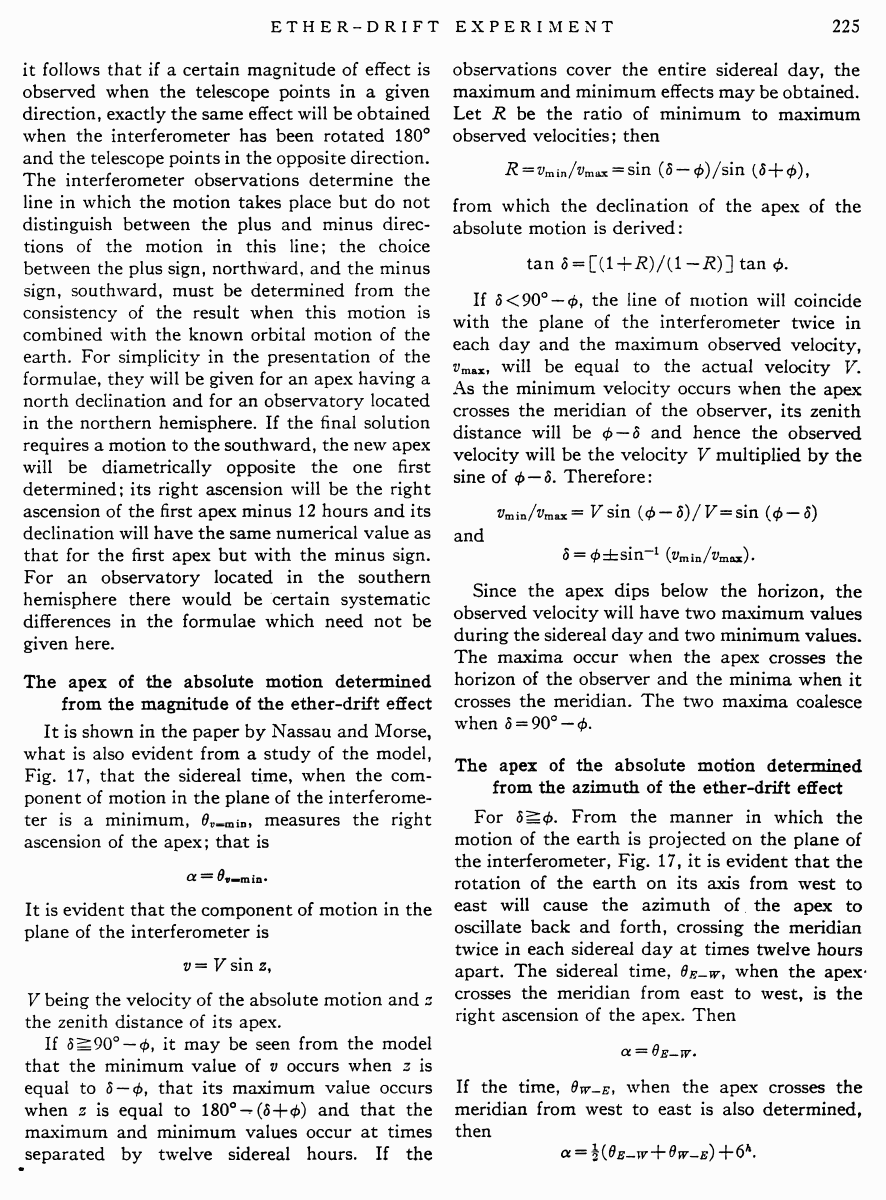# Miller D.C. The Ether-Drift Experiment and the Determination of the Absolute Motion of the Earth // Reviews of modern physics, Vol.5, July 1933

Start   PDF   <<<  Page 224   >>>203  204  205  206  207  208  209  210  211  212  213  214  215  216  217  218  219  220  221  222  223  224 225  226  227  228  229  230  231  232  233  234  235  236  237  238  239  240  241  242

Fig. 18. Model for studying the components of ether-drift.

azimuth variation, except insofar as they may be produced by one cause.

The model shown in Fig. 18, was prepared to assist in a study of the ether-drift effect in its astronomical relations. The large circular disk represents the plane of the interferometer which can be rotated around the inclined polar axis, bringing its plane into all the possible diurnal positions, corresponding to the Mount Wilson location. At the center of the disk is mounted a parallelogram whose sides can be made to represent any assumed values for the two components of the absolute motion, while the directions can be set as desired and the corresponding resultant will be reproduced. A small electric lamp is so supported that, as the inter

Fig. 19. Model illustrating the diurnal variation in the azimuth of the ether-drift.

ferometer is rotated around the polar axis and while the parallelogram remains stationary, the lamp casts the shadow of the resultant on the plane of the interferometer, showing how the azimuth of the drift varies with the time of day. The angle which the resultant makes with the plane can be observed and thus the variation in magnitude of the drift for the assumed motion is determined. A probable value for the cosmical component of motion having been selected, a single wire representing the resultant for any epoch is substituted for the parallelogram and the diurnal variations in azimuth and magnitude are studied. Three views of the model, Fig. 19, show how the azimuth swings to the west of north and then to the east, for the motion assumed.

It is evident from these models that the observed ether-drift effect would be very different for different resultant motions, as for different epochs, and that it would vary greatly in different latitudes. The conditions shown correspond approximately to the results here to be considered.

Solution for the absolute motion of the solar system

The point on the celestial sphere towards which the earth is moving because of its absolute motion is called the apex of its motion. This point is defined by its right ascension and declination, as is a star, and the formulae of practical astronomy are directly applicable to its determination from the interferometer observations. The theoretical consideration of the determination of the apex of the motion of the earth has been given in a paper by Professor J. J. Nassau and Professor P. M. Morse, which appeared in the Astrophysical Journal for March, 1927.11

Knowing the latitude of the observatory, φ, and the sidereal time, θ, of the observation, two independent determinations may be obtained for the right ascension, α, and for the declination, δ, of the apex of the earthÆs absolute motion, one determination from the observed velocity, V, and one from the azimuth, A, of the ether-drift effect. The ether-drift effect being a second order effect, periodic in each half turn of the apparatus,

11 J. J. Nassau and P. M. Morse, Astrophys. J. 65, 73 (1927).

it follows that if a certain magnitude of effect is observed when the telescope points in a given direction, exactly the same effect will be obtained when the interferometer has been rotated 180░ and the telescope points in the opposite direction. The interferometer observations determine the line in which the motion takes place but do not distinguish between the plus and minus directions of the motion in this line; the choice between the plus sign, northward, and the minus sign, southward, must be determined from the consistency of the result when this motion is combined with the known orbital motion of the earth. For simplicity in the presentation of the formulae, they will be given for an apex having a north declination and for an observatory located in the northern hemisphere. If the final solution requires a motion to the southward, the new apex will be diametrically opposite the one first determined; its right ascension will be the right ascension of the first apex minus 12 hours and its declination will have the same numerical value as that for the first apex but with the minus sign. For an observatory located in the southern hemisphere there would be certain systematic differences in the formulae which need not be given here.

The apex of the absolute motion determined from the magnitude of the ether-drift effect

It is shown in the paper by Nassau and Morse, what is also evident from a study of the model, Fig. 17, that the sidereal time, when the component of motion in the plane of the interferometer is a minimum, θv=min, measures the right ascension of the apex; that is

α = θv=min.

It is evident that the component of motion in the plane of the interferometer is

υ = V sin z,

V being the velocity of the absolute motion and z the zenith distance of its apex.

If δ ≥ 90░ û φ, it may be seen from the model that the minimum value of v occurs when z is equal to δ û φ, that its maximum value occurs when z is equal to 180░ û (δ +φ) and that the maximum and minimum values occur at times separated by twelve sidereal hours. If the

observations cover the entire sidereal day, the maximum and minimum effects may be obtained. Let R be the ratio of minimum to maximum observed velocities; then

R = vmin / vmax = sin (δ û φ) / sin (δ +φ),

from which the declination of the apex of the absolute motion is derived:

tan δ = [(1 +R) / (l û R)] tan φ.

If δ < 90░ ù φ, the line of motion will coincide with the plane of the interferometer twice in each day and the maximum observed velocity, vmax, will be equal to the actual velocity V. As the minimum velocity occurs when the apex crosses the meridian of the observer, its zenith distance will be φ û δ and hence the observed velocity will be the velocity V multiplied by the sine of φ û δ. Therefore:

vmin / vmax = V sin (φ û δ) /V = sin (φ û δ)

and

## δ = φ ▒ sin û1 (vmin/vmax).

Since the apex dips below the horizon, the observed velocity will have two maximum values during the sidereal day and two minimum values. The maxima occur when the apex crosses the horizon of the observer and the minima when it crosses the meridian. The two maxima coalesce when δ = 90░ û φ.

The apex of the absolute motion determined from the azimuth of the ether-drift effect

For δ ≥ φ. From the manner in which the motion of the earth is projected on the plane of the interferometer, Fig. 17, it is evident that the rotation of the earth on its axis from west to east will cause the azimuth of the apex to oscillate back and forth, crossing the meridian twice in each sidereal day at times twelve hours apart. The sidereal time, θEûW, when the apex crosses the meridian from east to west, is the right ascension of the apex. Then

α = θEûW

If the time, θWùE, when the apex crosses the meridian from west to east is also determined, then

α = (1/2)(θEûW WùE) +6h.